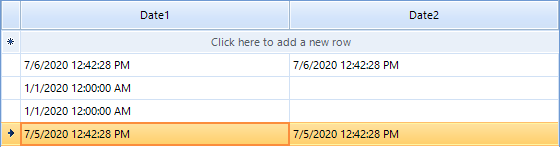# Set NullValue for each row of GridViewDateTimeColumn

1. ##### özer22 posts
Member since:
Jun 2020

Posted 02 Jul Link to this post

Hi.

I take the table on a web page with C # and Selenium and show it in radGridView.
I add a GridViewCheckBoxColumn before the first column of each row, and I add GridViewDateTimeColumn and GridViewComboBoxColumn after the last column.
I want to equalize the initial value of the DatePicker object in GridViewDateTimeColumn to the value in column 7 of the row where it is located.

However, the initial value of DatePickers in all rows is equal to the value in the 7th column of the last row.

Here is my code:

`01.``public` `void` `FillRadGridView(List<IWebElement> rows, List<IWebElement> columns,  ``int` `rowCount, ``int` `columnCount, RadGridView rgv)`
`02.``        ``{`
`03.`
`04.``            ``List<Visit> lst = ``new` `List<Visit>();`
`05.``            ``Visit v = ``new` `Visit();`
`06.``            ``for` `(``int` `i = 0; i < rowCount; i++)`
`07.``            ``{`
`08.``                ``for` `(``int` `j = 1; j <= columnCount; j++)`
`09.``                ``{`
`10.`
`11.``                    ``v.Tckn = columns.Text;`
`12.``                    ``v.AdSoyad = columns.Text;`
`13.``                    ``v.Vaka = columns.Text;`
`14.``                    ``v.RaporTakipNo = columns.Text;`
`15.``                    ``v.RaporSiraNo = columns.Text;`
`16.``                    ``v.RaporBaslamaTarihi = DateTime.Parse(columns.Text).ToShortDateString();`
`17.``                    ``v.RaporBitisTarihi = DateTime.Parse(columns.Text).AddDays(-1).ToShortDateString();`
`18.``                    ``v.IsBasiKontrolTarihi = DateTime.Parse(columns.Text).ToShortDateString();`
`19.``                    ``v.CezaDurumu = columns.Text;`
`20.`
`21.``                ``}`
`22.``                ``lst.Add(v);`
`23.``            ``}`
`24.``            ``rgv.Columns.Clear();`
`25.`
`26.``            ``GridViewCheckBoxColumn checkBoxColumn = ``new` `GridViewCheckBoxColumn();`
`27.``            ``checkBoxColumn.DataType = ``typeof``(``int``);`
`28.``            ``checkBoxColumn.Name = ``"ColumnSelect"``;`
`29.``            ``checkBoxColumn.FieldName = ``"select"``;`
`30.``            ``checkBoxColumn.HeaderText = ``"Seç"``;`
`31.``            ``rgv.Columns.Add(checkBoxColumn);`
`32.`
`33.``            ``rgv.DataSource = lst;`
`34.``            ``AddColumnToRgv(rgv);`
`35.``            `
`36.``        ``}`

2. ##### Dess | Tech Support Engineer, Sr.3903 posts

Posted 06 Jul Link to this post

Hello, özer,

According to the provided information, it is not clear enough what is the Telerik product that you are currently using since this is the forum related to the Telerik UI for WinForms suite but you mentioned "web page".  I am providing an answer regarding the Telerik WinForms product. In case you are using another product, feel free to post your question in the relevant forum: https://www.telerik.com/forums

The provided information is also not enough to get full understanding of the precise case and the setup that you have. However, I will try to suggest a possible solution.

If you have an assigned value to a cell in GridViewDateTimeColumn, the cell is expected to show this value. Note that a GridViewDateTimeColumn offers the NullValue property. Thus, you specify what date value to be displayed within the cell if you have DBNull.Value or null

```            GridViewDateTimeColumn col1 = new GridViewDateTimeColumn("Date1");
col1.NullValue = new DateTime(2020, 1, 1);

GridViewDateTimeColumn col2 = new GridViewDateTimeColumn("Date2");